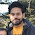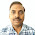## 3 Phase Induction Motors Related ( MCQ ) Objective Questions with Answers

 Which of the following statements about a three-phase squirrel-cage induction motor are false?
(a) It has no external electrical connections to its rotor
(b) A three-phase supply is connected to its stator
(c) A magnetic flux which alternates is produced
(d) It is cheap, robust and requires little or no skilled maintenance

(1) A,B,C only
(2) C and D only
(3) C only
(4) None of the above
(5) B only

 Which of the following statements about a three-phase induction motor are false?
(a) The speed of rotation of the magnetic field is called the synchronous speed
(b) A three-phase supply connected to the rotor produces a rotating magnetic field
(c) The rotating magnetic field has a constant speed and constant magnitude
(d) It is essentially a constant speed type machine

(1) C only
(2) B only
(3) A only
(4) All of the above
(5) A and C only

 Which of the following statements is false when referring to a three-phase induction motor?
(a) The synchronous speed is half the supply frequency when it has four poles
(b) In a 2-pole machine, the synchronous speed is equal to the supply frequency
(c) If the number of poles is increased, the synchronous speed is reduced
(d) The synchronous speed is inversely proportional to the number of poles

(1) All of the above
(2) D only
(3) B only
(4) None of the above
(5) A and C only

 A 4-pole three-phase induction motor has a synchronous speed of 25 rev/s. The frequency of the supply to the stator is:
(a) 50 Hz
(b) 100 Hz
(c) 25 Hz
(d) 12.5 Hz

 In a three-phase induction motor. Which of the following statements are false?
(a) The slip speed is the synchronous speed minus the rotor speed
(b) As the rotor is loaded, the slip decreases
(c) The frequency of induced rotor e.m.f.’s increases with load on the rotor
(d) The torque on the rotor is due to the interaction of magnetic fields

(1) All of the above
(2) C only
(3) B only
(4) A and C only
(5) B and D only

 In a three-phase induction motor. Which of the following statements are false?
(a) If the rotor is running at synchronous speed, there is no torque on the rotor
(b) If the number of poles on the stator is doubled, the synchronous speed is halved
(c) At no-load, the rotor speed is very nearly equal to the synchronous speed
(d) The direction of rotation of the rotor is opposite to the direction of rotation of the magnetic field to give maximum current induced in the rotor bars

(1) A, B , C
(2) C only
(3) B only
(4) A and C only
(5) D only

 The slip speed of an induction motor may be defined as the:
(a) Number of pairs of poles ÷ frequency
(b) Rotor speed - synchronous speed
(c) Rotor speed + synchronous speed
(d) Synchronous speed  - rotor speed

 The slip speed of an induction motor depends upon:
(a) Armature current
(b) Supply voltage
(d) Eddy currents

 The starting torque of a simple squirrel-cage motor is:
(a) Low
(b) Increases as rotor current rises
(c) Decreases as rotor current rises
(d) High

 The slip speed of an induction motor:
(a) is zero until the rotor moves and then rises slightly
(b) is 100 per cent until the rotor moves and then decreases slightly
(c) is 100 per cent until the rotor moves and then falls to a low value
(d) is zero until the rotor moves and then rises to 100 per cent

7:44 AM

1.Dear Respected sir Kindly send us more questions at my email I'd
babar685@gmail.com

2.Dear sir u are too good

3.Please sent email id mohit2511619@gmail.com

4.Very much useful

5.Dear Sir plz kindly request you to send more questions at my mail shreelakshmi050@gmail.com... Its very much useful for all exams..
Thank u sir

1.Expected and logic thinking bits.good

6.Dear sir,
as per my knowledge synchronous speed is inversely proportional to number of poles
Ns = 120f/p
But you have given in the answer it is false statement for question -3, so plz clarify the same.
Thank you

1.Yes you are correct

7.Question no 3:- Ns=120f/P synchronous speed inversely proportional to number of poles it's coreect.

8.You have to search most false statement.

9.As per question, I think, (3) B only should be right answer.

10.Questions are really good. But answers are not correct.